Function Repository Resource:

# GeometricSceneConstructor

Create an interface for constructing a GeometricScene

Contributed by: Wolfram|Alpha Math Team (Jeremy Stratton-Smith)
 ResourceFunction["GeometricSceneConstructor"][] creates an interactive tool for generating a GeometricScene.

## Details

ResourceFunction["GeometricSceneConstructor"] creates an interactive interface that allows users to create primitives and relations among them and then produces the corresponding GeometricScene or RandomInstance expression without the user having to build the code from the ground up.
The interface is broken up into two components, "Figures" and "Relations":
 Figures area for adding primitives such as Line,Circle,Triangle, etc. Relations area for adding relationships among the defined primitives such as "Parallel", "Inside", etc.
Figures are determined by symbols entered under the "Specifications" column, which accepts symbols and numbers in some cases (radius and angle for example). Additional properties of a given figure can be chosen from the fields under the "Properties" column while the "Styles" column allows users to customize the appearance of particular figures in the output.
To add a relation, the user must first enter one or more figures and then select them from the dialog accessed via the select figures button. Once the desired figures are selected, clicking select relations opens another dialog which allows for the particular kinds of relations available for the selected figures to be chosen.
Clicking Print GeometricScene will print out the symbolic object corresponding to the description provided while clicking Visualize GeometricScene will print a cell with and evaluate it to show a visualization of the scene.
Note that some properties and relations are mutually exclusive (i.e. if one is selected, selecting another for the same figure/relation will replace that selection with a different option).

## Examples

### Basic Examples (2)

Create an interface for constructing GeometricScene expressions:

 In:=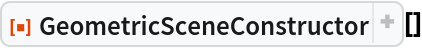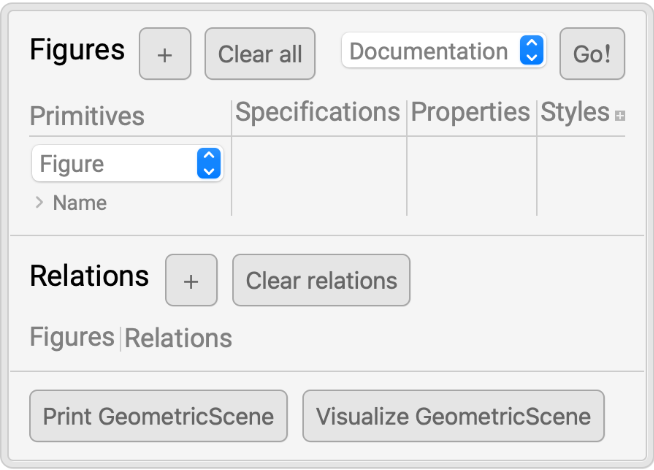Add some figures, specifying the points and constants as needed: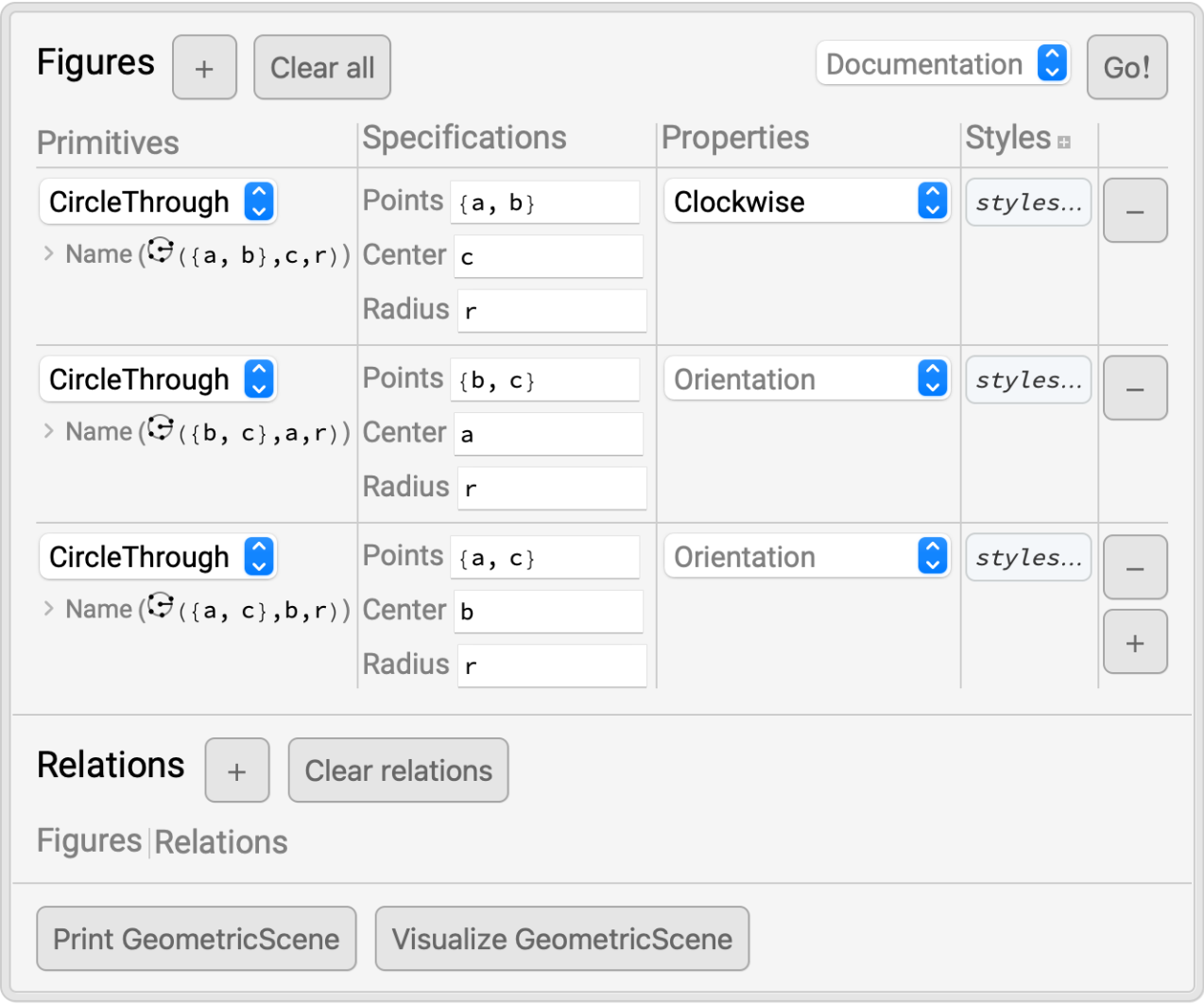Click Visualize GeometricScene to create and evaluate code that generates an instance of the scene. Even without any relations, we are able to build interesting scenes:

 In:=Out=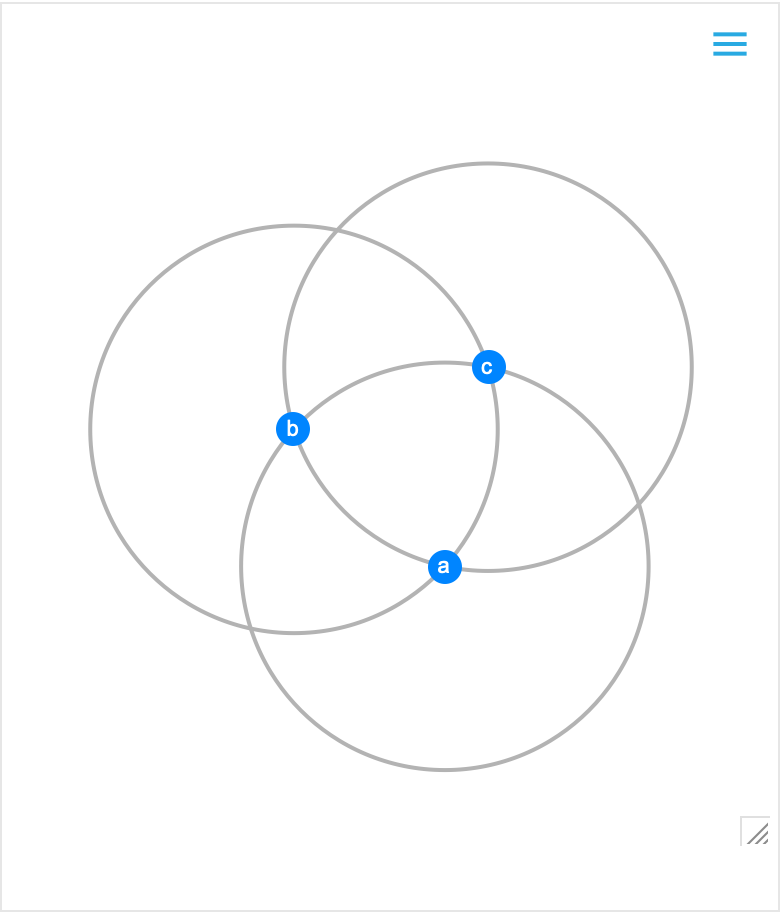Construct famous scenes like Thales's theorem:

 In:=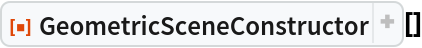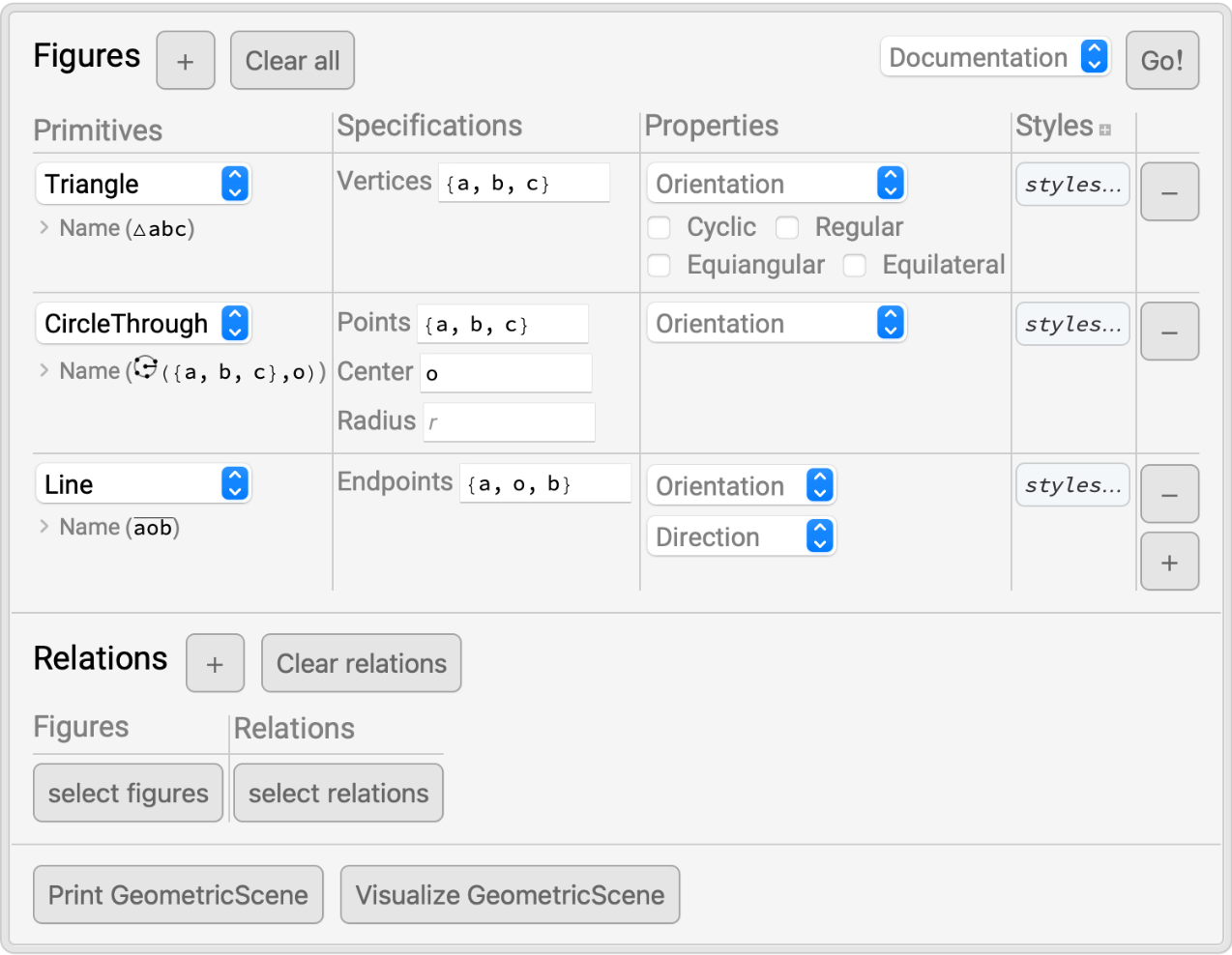In:=Out=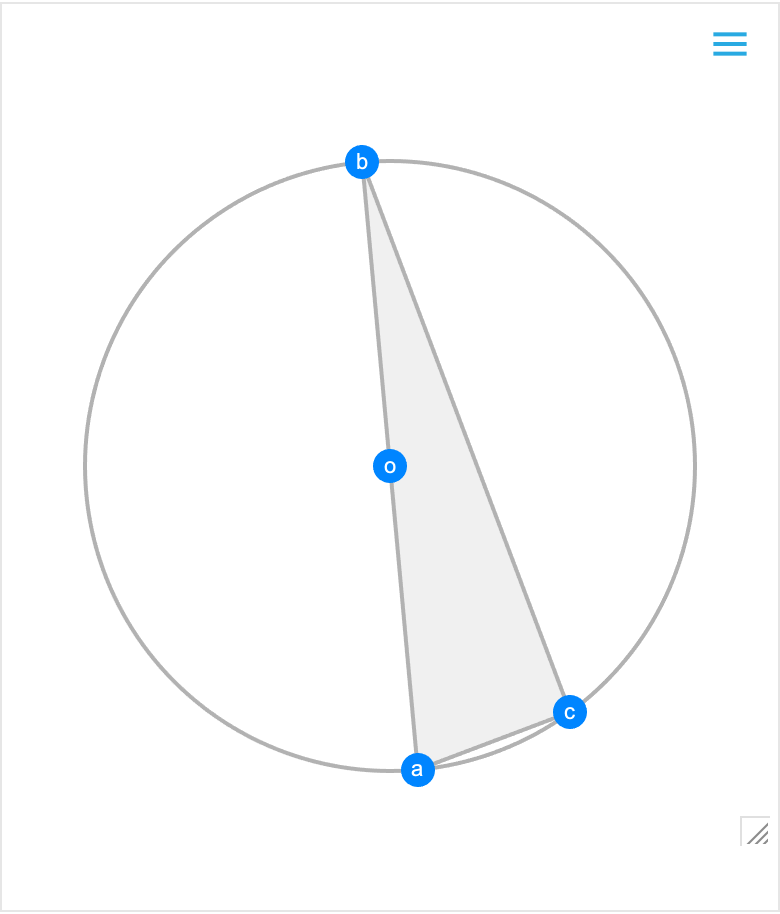### Scope (3)

Use the "Properties" column to state properties of individual figures:

 In:=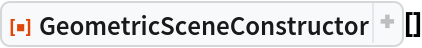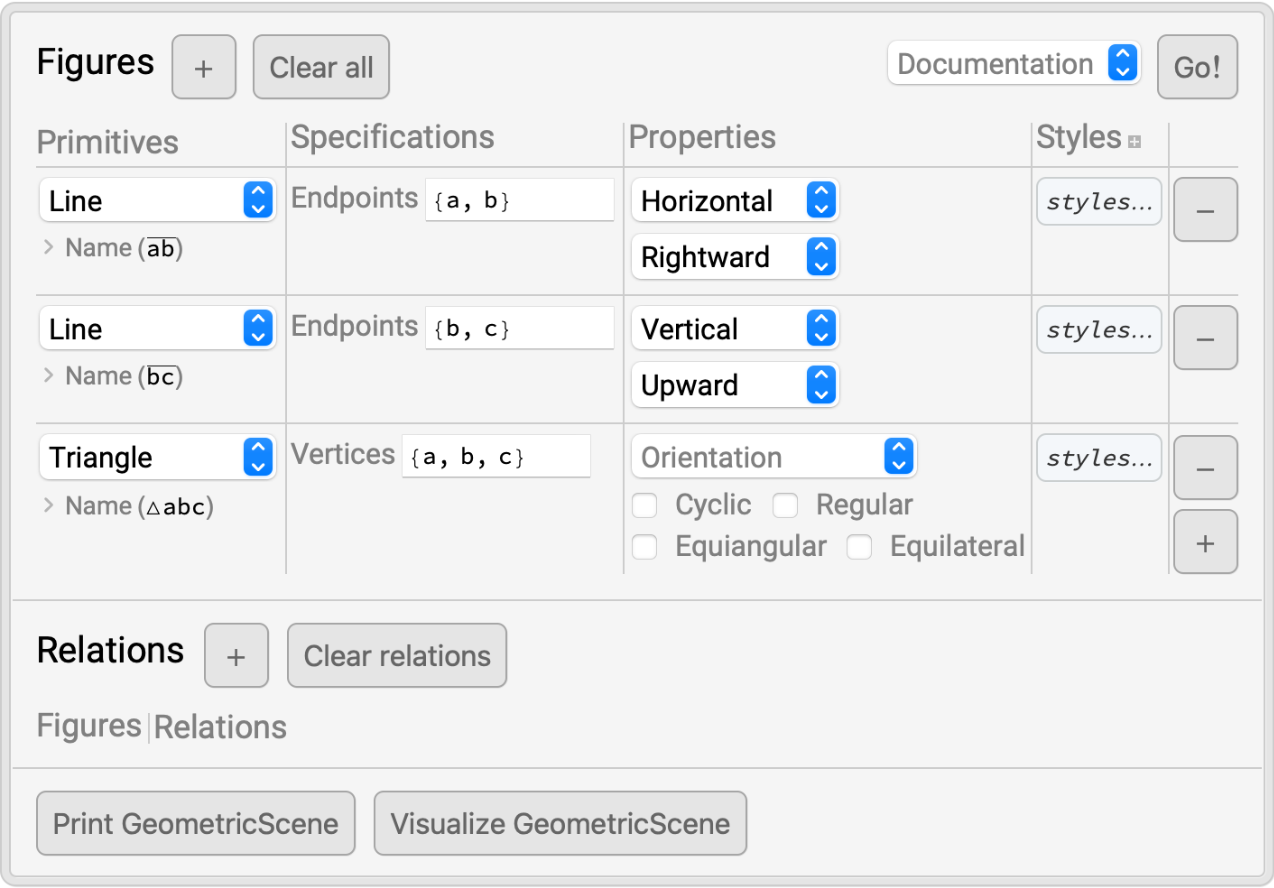In:=Out=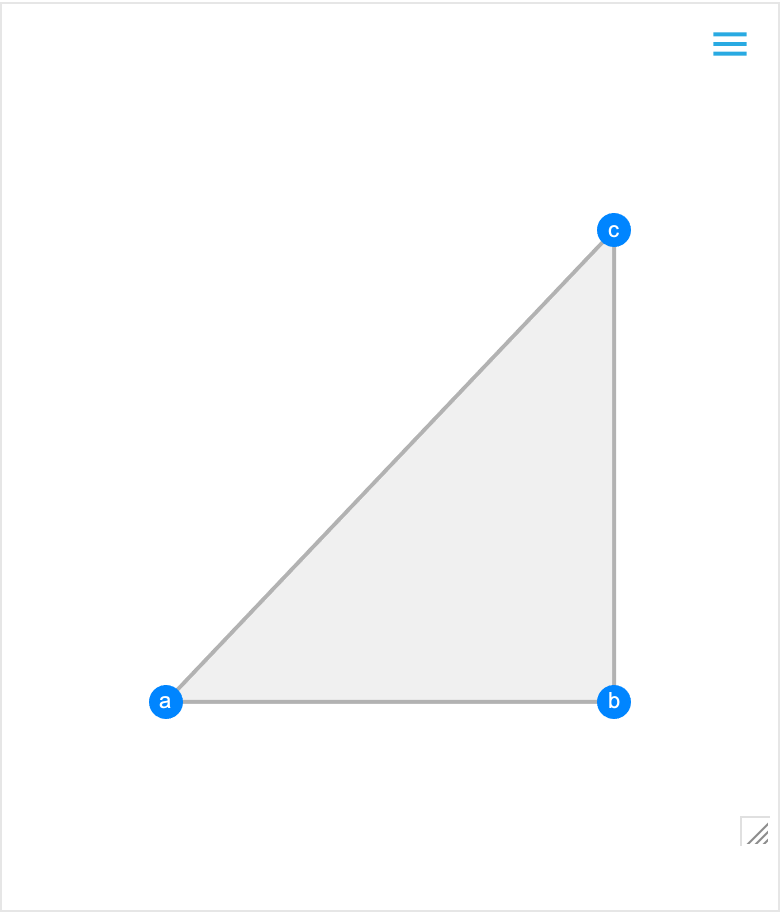Construct scenes with constraints or conditions on the figures by introducing relations:

 In:=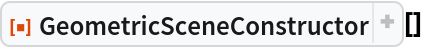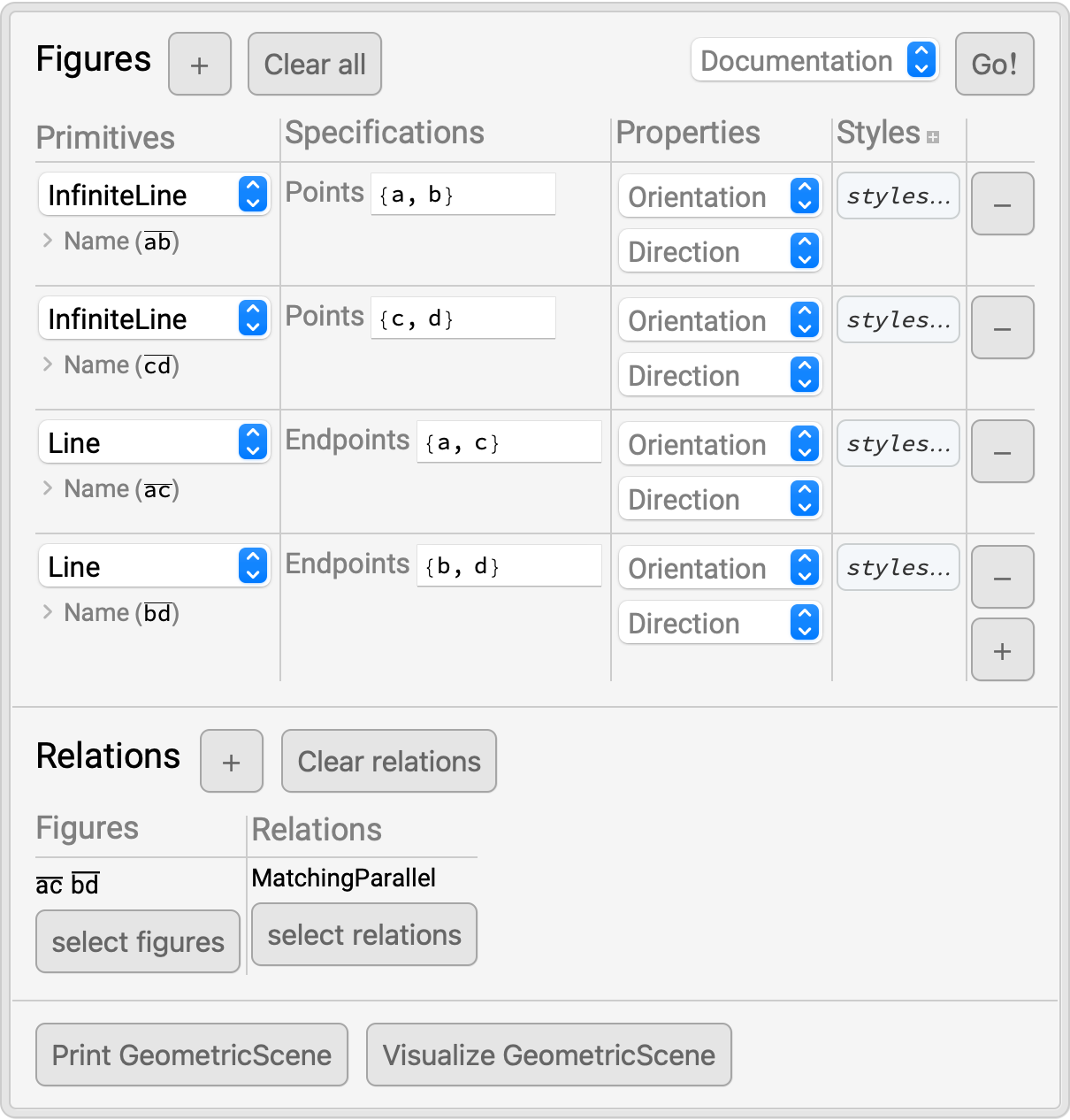In:=Out=Click on the "Styles" column header to modify the appearance of elements in your scene:

 In:=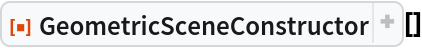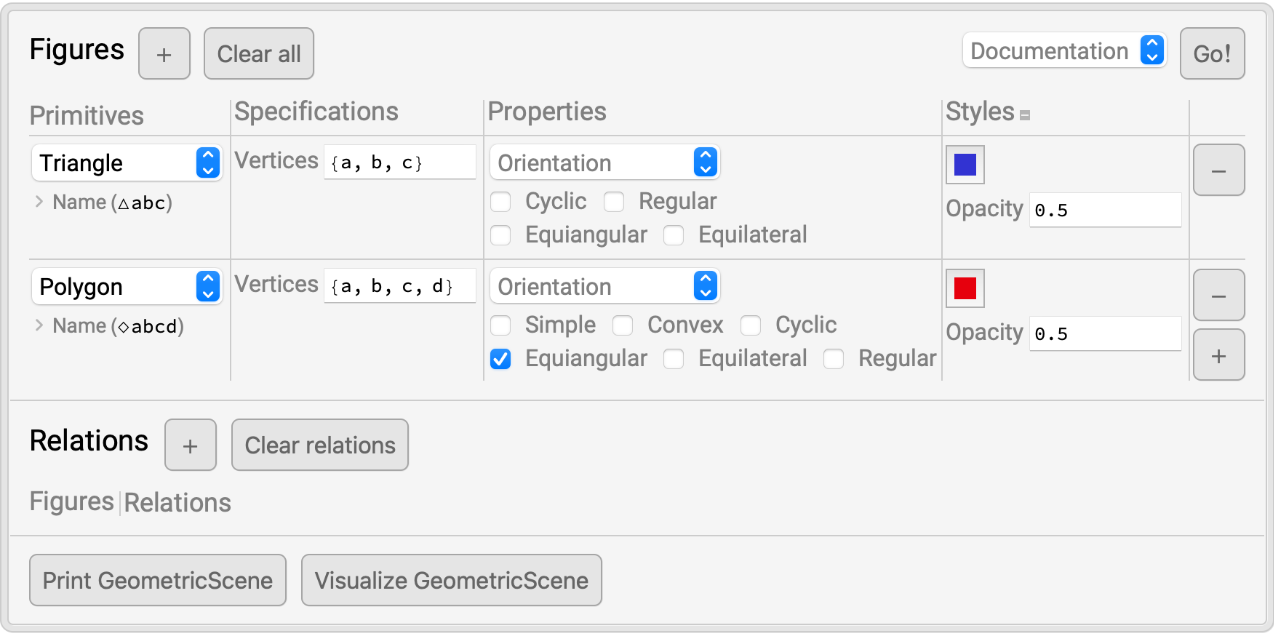In:=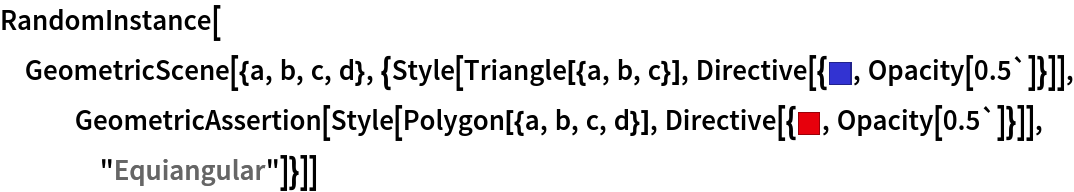Out=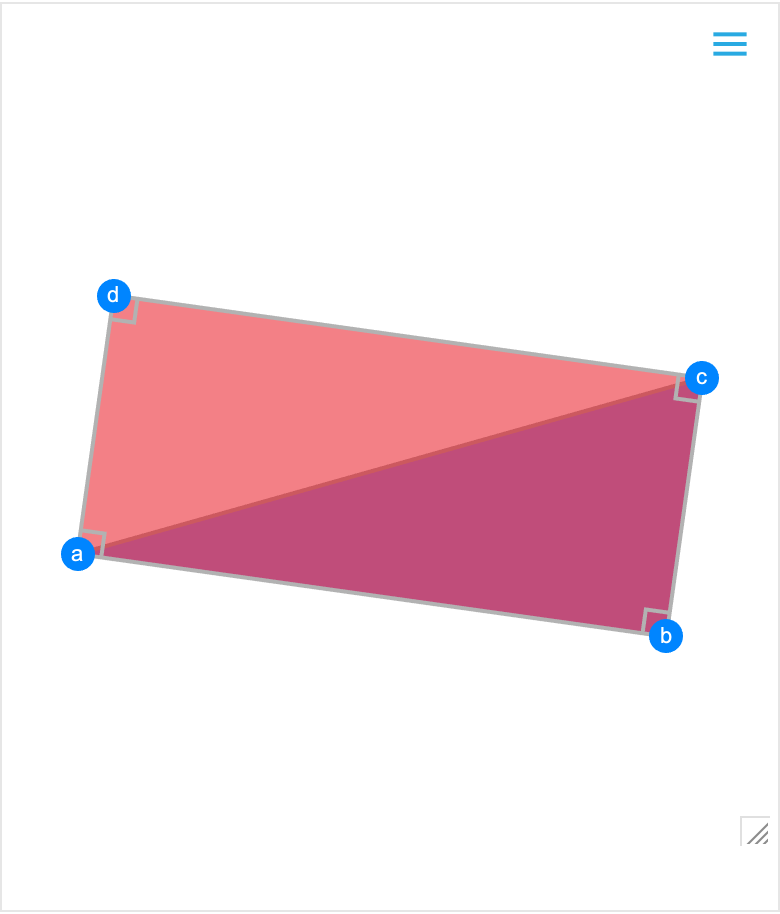### Properties and Relations (2)

Construct a simple scene and visualize it:

 In:=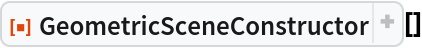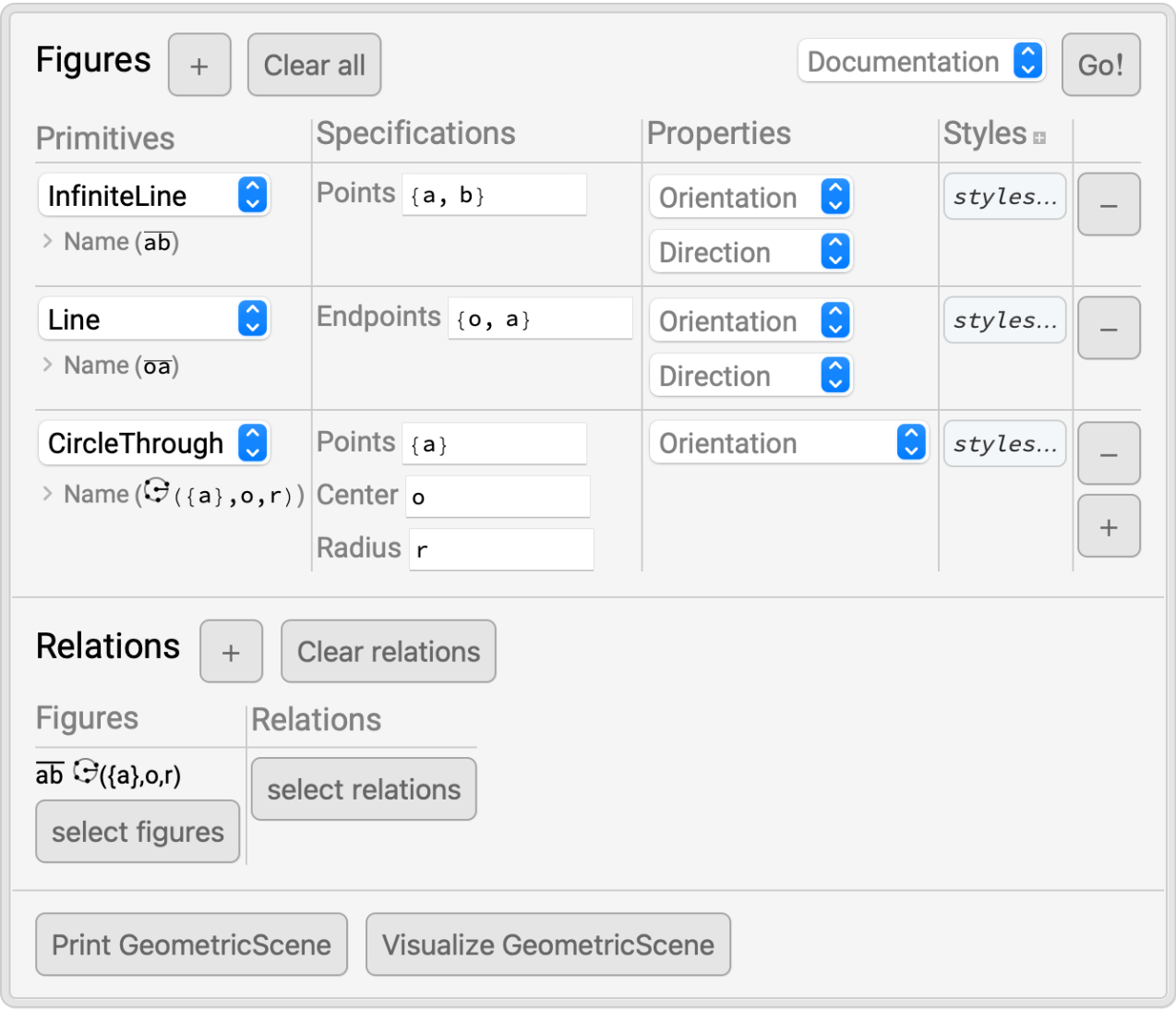In:=Out=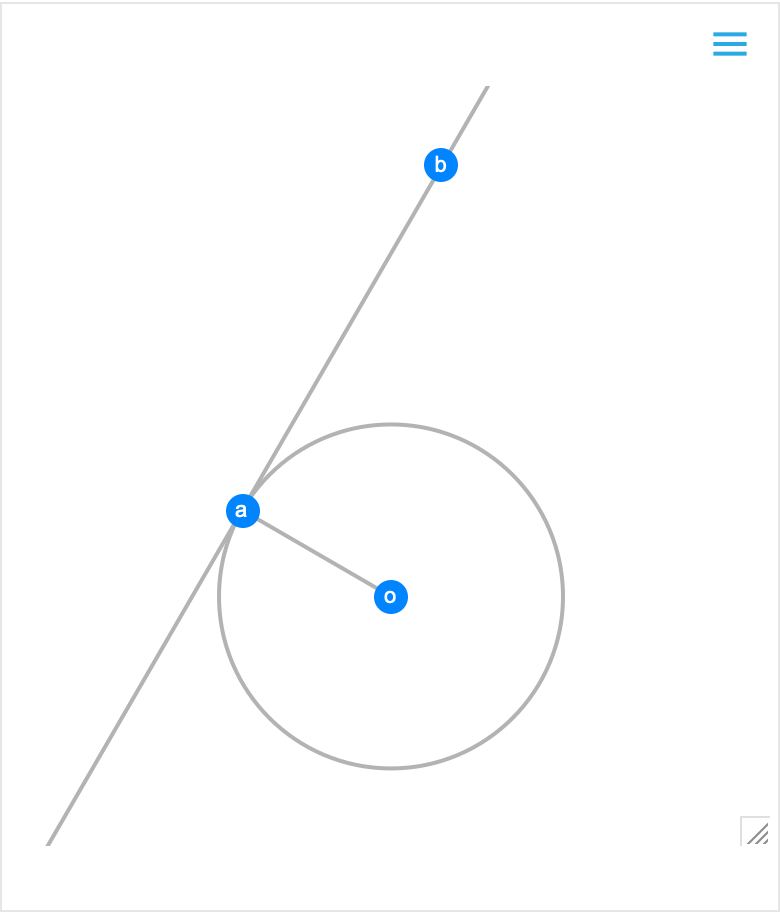Then use FindGeometricConjectures to draw conclusions from the scene:

 In:=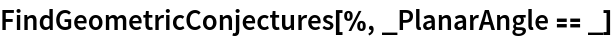Out=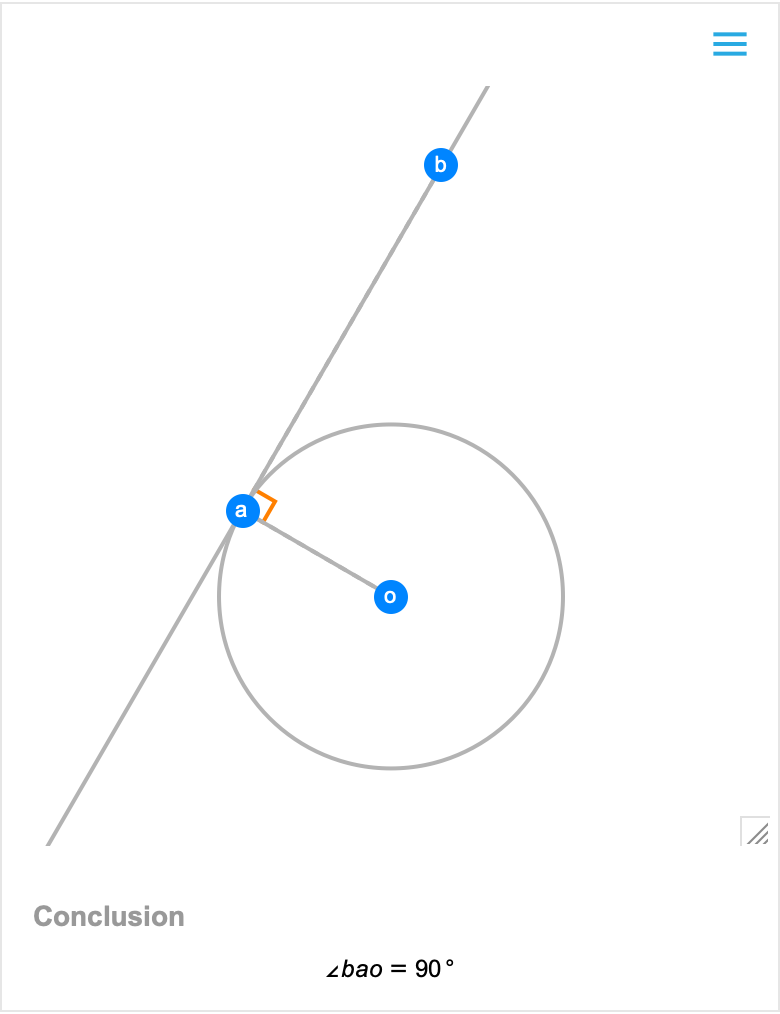Construct a geometric scene with a numerical value for an angle:

 In:=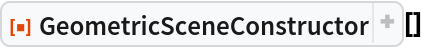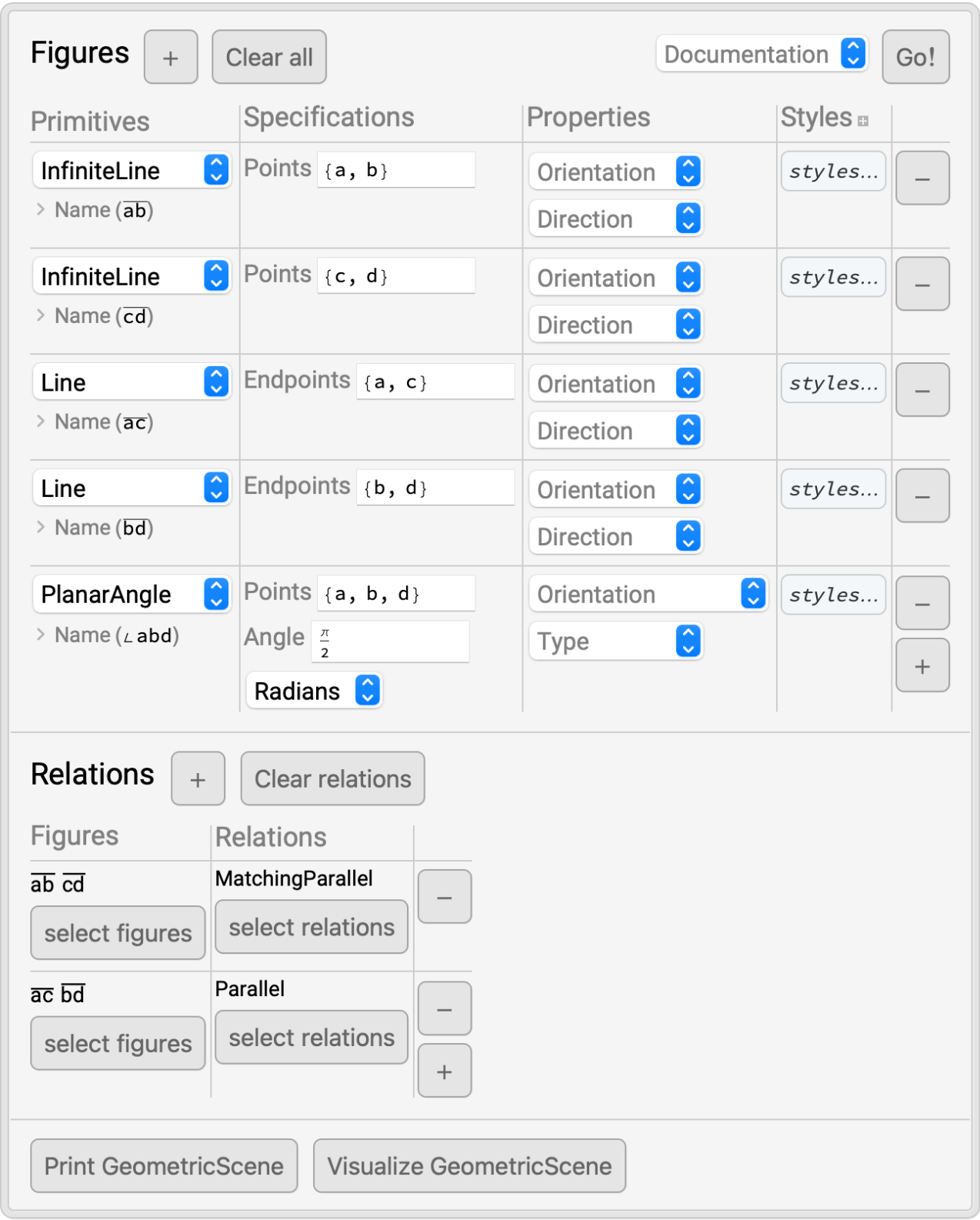In:=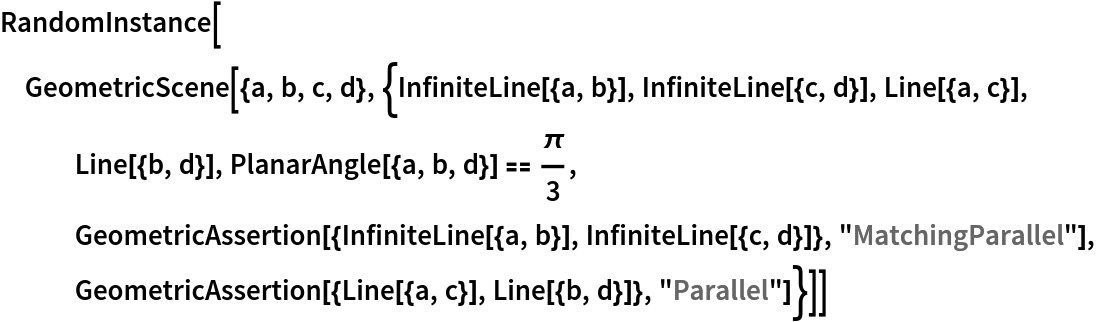Out=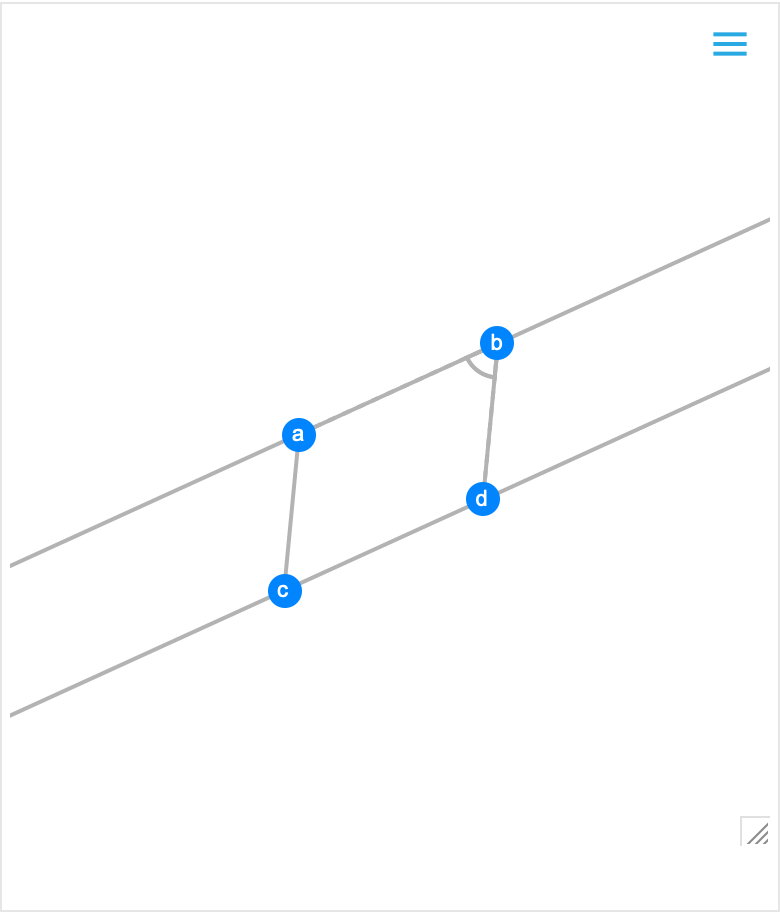Use the resource function GeometricSolve to compute the numerical value of another angle from the scene and confirm that it is the same value as the opposite angle:

 In:=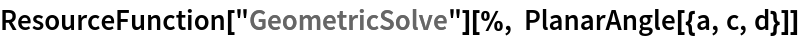Out=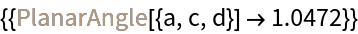In:=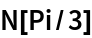Out=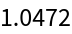## Publisher

Wolfram|Alpha Math Team

## Version History

• 2.0.0 – 23 March 2023
• 1.0.1 – 19 April 2022
• 1.0.0 – 12 April 2022

## Author Notes

To view the full source code for GeometricSceneConstructor, evaluate the following:

 In:=